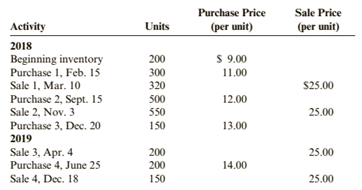Chapter 6, Problem 73APSA### Cornerstones of Financial Accounti...

4th Edition
Jay Rich + 1 other
ISBN: 9781337690881

#### Solutions

Chapter
Section### Cornerstones of Financial Accounti...

4th Edition
Jay Rich + 1 other
ISBN: 9781337690881
Textbook Problem
187 views

# ( Appendix 6B) Inventory Costing MethodsJet Black Products uses a periodic inventory system. For 2018 and 2019, Jet Black has the following data:All purchases and sales are for cash.Required:1. Compute cost of goods sold, the cost of ending inventory, and gross margin for each year using FIFO.2. Compute cost of goods sold, the cost of ending inventory, and gross margin for each year using LIFO.3. Compute cost of goods sold, the cost of ending inventory, and gross margin for each year using the average cost method. ( Note: Use four decimal places for per unit calculations and round all other numbers to the nearest dollar.)4. CONCEPTUAL CONNECTION Which method would result in the lowest amount paid for taxes?5. CONCEPTUAL CONNECTION Which method produces the most realistic amount for income? For inventory? Explain your answer.6. CONCEPTUAL CONNECTION What is the effect of purchases made later in the year on the gross margin when LIFO is employed? When FIFO is employed? Be sure to explain why any differences occur.7. CONCEPTUAL CONNECTION If you worked Problem 6-68A, compare your answers. What are the differences? Be sure to explain why any differences occurred.

To determine

(a)

Inventory costing methods:

FIFO, LIFO and average cost method, are those method which used for calculation of closing inventory and cost of goods sold.

The cost of ending inventory, the cost of goods sold and gross margin using the FIFO.

Explanation

The given information for the year 2018 is as follows:

Total available units are:

Opening inventory=200 units @ $9 each Purchases=300 units @$11 eachPurchases=500 units @ $12 eachPurchases=150 units @$13 each

Total Purchased units = 950

Total available quantity = 200+950= 1150 units

Sales=320+550 units=870 units

Closing inventory =Beginning inventory + PurchaseSales=200+950870=280 units

The given information for the year 2019 is as follows:

Total available units are:

Opening inventory=130 units @ $12 each=150 units @$13 each

Purchases=200 units @ $14 each Total Purchased units = 200 Total available quantity = 280+200= 480 units Sales=200+150 units=350 units Closing inventory =Beginning inventory + PurchaseSales=280+200350=130 units Calculation of Closing Inventory as per FIFO Method: Under this method, which material purchased first, issued first for production. However closing inventory includes last purchased materials in stock. Due to latest purchase in closing inventory, higher value of latest purchase effects cost of goods sold as lower and profit margin will be high. As in the periodic system, no updated records are available. So, the cost of goods sold will be computed by subtracting the cost of goods available for sale and cost of ending inventory. The method followed here is FIFO in which the closing inventory of 280 units will be valued at last price of purchases i.e.$12 and $13 in the year 2018 and 130 units will be valued at last price of purchases i.e.$14 in the year 2019 as in this method the sale is made from first purchase which leads to last purchase to be left on the inventory

To determine

(b)

Inventory costing methods:

FIFO, LIFO and average cost method, are those method which used for calculation of closing inventory and cost of goods sold.

The cost of ending inventory, the cost of goods sold and gross margin using the LIFO.

To determine

(c)

Inventory costing methods:

FIFO, LIFO and average cost method, are those method which used for calculation of closing inventory and cost of goods sold.

The cost of ending inventory, the cost of goods sold and gross margin using the average cost method.

To determine

(d)

Inventory costing methods:

FIFO, LIFO and average cost method, are those method which used for calculation of closing inventory and cost of goods sold.

The method for determining the lowest amount paid for tax.

To determine

(e)

Inventory costing methods:

FIFO, LIFO and average cost method, are those method which used for calculation of closing inventory and cost of goods sold.

The method for determining the most realistic amount for inventory.

To determine

(f)

Gross profit margin ratio:

The gross margin ratio is a type of profitability ratio which is used to measure the returns and earning after direct expenses and compute the ratio in respect to the sales of the business.

Inventory Turnover ratio:

The ratio which measures the efficiency of the company in managing their inventory by diving the cost of goods sold by the average inventory.

The effect of purchases made later in the year on the gross margin when LIFO and FIFO is employed.

To determine

(g)

Inventory costing methods:

FIFO, LIFO and average cost method, are those method which used for calculation of closing inventory and cost of goods sold.

The difference of the results in two same answers.

### Still sussing out bartleby?

Check out a sample textbook solution.

See a sample solution

#### The Solution to Your Study Problems

Bartleby provides explanations to thousands of textbook problems written by our experts, many with advanced degrees!

Get Started